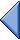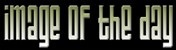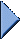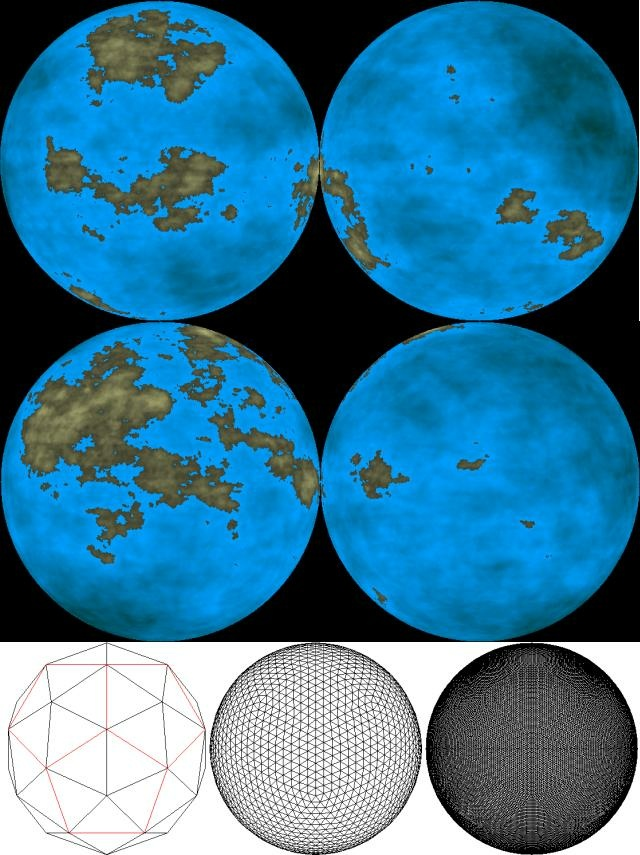Submitted by , posted on 17 December 2001Image Description, byThis is from a terrain generator that I've been working on in the last couple of weeks. So you may ask what's the big deal? I know there are SEVERAL random terrain generators out there but the problem with them is that they generally cannot generate a full-sized planet. They are generally created on a square or rectangular grid and therefor discontinous at the borders. So here goes. The way I do it is to generate a sphere from 12 connected pentagons (like a tetrahedron, but with pentagons instead of triangles). This gives me 12*5=60 triangles to work with as a basic sphere. You'll see this in the bottom left picture. I outlined three of the pentagons with red. To increase the poly count in the sphere, I simply split each of these triangles into four additional triangles. The middle and left picture contains 3840 and 61440 polys respectively. This is done blindingly fast and doesn't seem to impact the generation (which I'll get to just now) much. I store the vertices and the triangles in two arrays; the triangles as a triangles list with each triangle having three indices referring to its three vertices PLUS three indices referring to it's neighbouring triangles (might be important for some reason like triangle strips or something). Another reason I used 12 pentagons was to have all of the triangles in the final sphere model almost the same size. Tetrahedrons as base models normally have a wide size range, especially at high triangle counts. The terrain generation is very simple: I form a random plane at a random distance perpendicular to some random vector eminating from the centre of the sphere. This distance will of course be between 0 and the radius of the sphere. Then I go through the vertex list and determine for each vertex whether the vertex is in front of or behind the plane (simple dot product calculation). The vertices on the one side of the plane is raised whereas those on the other side are left as they are. I say here raised but for every vertex I have also included a height property and this I increase or decrease, I don't actually alter the spatial coordinate of the vertex (this can be done at a later stage). Another thing to keep the continents balanced is that every iteration I change which side of the plane heights are altered, i.e. Iteration 1: front is raised, iteration 2: back is raised, etc. Otherwise you end up with Himalayas on one side of your planet and the Abyss on the other. The two half-spheres in the top of the picture are the results. You can see that the terrain is continuous all over the surface of the planet. The spheres I used consisted of 245 760 triangles. I ran the program on a PIII 600MHz machine (no 3D yet) for a 1000 iterations (top) and 2000 iterations (bottom). The first terrain took about 4.5 minutes and the second 9 minutes. There seems to be a linear increase in time with an increase in one of the variables (number of polygons and number of iterations). You'll also see that with increasing number of iterations some of the sharp "cliffs" are removed. The smoothness of the terrain will improve with increasing number of iterations. Possible applications I can think of at the moment would be planet generation for a planet-wide strategy game or something like that. Anyway, if anybody wants the code, just mail me. There's still some minor alterations that I'd like to make and documentation to write. (all code in C++). Rho